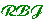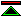# The Axiomatic Method

 1. Invented By The Greeks The ancient Greeks devised the axiomatic method as a systematic way of organising the demonstrative or deductive reasoning which they considered essential to establish mathematical propositions. Key features include: definitions, postulates and common notions as starting points from which the propositions of the subject are derived. Key defects are the lack of formality, the intuitive nature of derivation, and hence the danger of hidden premises. 2. Formalised as First Order Logic In modern times demonstrative reasoning is formalised in (among many systems) first order logic. The precise formulation of first order logic reflects a modern view of the axiomatic method and also makes it easier to see how the method falls short of the needs of modern mathematics. Logical axioms correspond to common notions, non-logical axioms correspond to postulates, and primitive definitions to undefined logical constants. 3. Foundational Improvements During most of this century mathematics has been built on the foundation of first order set theory. This can be seen as an evolution of the axiomatic method, addressing more effectively some of the original concerns, and providing a better platform for modern mathematics. The foundational approach involves a strict axiomatic treatment of the theory of sets, which establishes an ontological framework within which all other mathematical theories can be introduced by definitions rather than axioms. The benefits of this are: Definitions are logically safer than axioms, since they cannot introduce inconsistency. In the ontological framework of set theory it is possible to reason about the relationships between different mathematical structures (e.g. homomorphisms between groups). This cannot be done using an axiomatisation of group theory, in which the domain of discourse consists of a single group. 4. Category Theoretic Reservations Some modern approaches to mathematics, notable the category theoretic approach, are not ideally supported by set theoretic foundations and suggest the need for further liberalisation of the cannons of demonstrative reasoning. Because of the risk of inconsistency highlighted by paradoxes such as Russell's paradox, not all the sets one might wish to see are available in classical set theory. It turns out that as mathematics becomes more abstract many of the structures which one might wish to reason about just don't exist in this context. For example, mathematical groups are related among themselves by homomorphisms, and we may wish to consider the structure of this network of homomorphisms and relate this structure to others, perhaps the homomorphisms between fields. These collections of homomorphisms are examples of another kind of structure called a category. Unfortunately, the collection of group homomorphisms in Zermelo-Fraenkel set theory is not itself a set, and so the categories we are interested in relating simply don't exist in this context.©created 1996/10/27, modified 1997/6/7